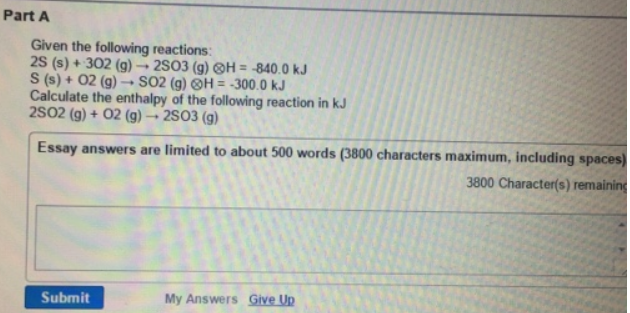# Problem: Given the following reactions: 2S (s) + 3O2 (g) → 2SO3 (g)  H = -840.0 kJ S (s) + O2 (g) → SO2 (g)        H = -300.0 kJCalculate the enthalpy of the following reaction in kJ 2SO2 (g) + O2 (g) → 2SO3 (g)

###### FREE Expert Solution
91% (84 ratings)###### Problem Details

Given the following reactions:

2S (s) + 3O2 (g) → 2SO3 (g)  H = -840.0 kJ

S (s) + O2 (g) → SO2 (g)        H = -300.0 kJ

Calculate the enthalpy of the following reaction in kJ

2SO2 (g) + O2 (g) → 2SO3 (g)